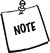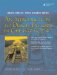# Pointers and Memory Access

C and C++ distinguish themselves from many other languages by permitting direct access to memory through the use of pointers. This section explains the basic pointer operations and modifiers, and introduces dynamic memory usage. Pointers can seem complicated at first. We discuss pointer use and misuse in more detail in Chapter 22.

1.12.1. The Unary Operators & and *

A variable is an object with a name recognized by the compiler. A variable's name can be used as if it is the object itself. For example, if we say:

```int x = 5;
```

we can use x to stand for the integer object whose value is 5, and we can manipulate the integer object directly through the name x. For example:

```++x ; // symbol x now refers to an integer with value 6
```

An object (in the most general sense) is a chunk of memory that can hold data. Each object has a memory address (where the data begins). The unary & operator, also known as the address-of operator, when applied to any object, returns the memory address of that object. For example, &x returns the memory address of x.

An object that holds the memory address of another object is called a pointer. We say that the pointer points to the object at the stored memory address.

```int* y = &x ;
```

In this example, y points to the integer x. The asterisk * following the int indicates that y is a pointer to int. Here we have initialized the int pointer y to the address of the int variable x. One of the powerful features of pointers is that, subject to rules that we will explore shortly, it is possible for a pointer of one type to hold the address of an object of a different (but related) type.

Zero (0), often represented by the macro NULL in C programs, is a special value that can be legally assigned to a pointer, usually when it is being initialized (or re-initialized). 0 is not the address of any object. A pointer that stores the value 0 is called a null pointer. Stroustrup recommends the use of 0 rather than the macro NULL in C++ programs.

A pointer to a simple type uses exactly the same amount of memory as a pointer to a large complicated object. That size is usually the same as sizeof(int) on that machine.

The unary * operator, also known as the dereference operator, when applied to a non-null pointer returns the object at the address stored by the pointer.The symbol * is used in two different ways in connection with pointers: It can serve as a type modifier in a pointer variable definition. It can be used as the dereference operator.Dereferencing a null or uninitialized pointer causes a run-time error, usually a segmentation fault or, in Windows, a General Protection Fault (GPF). It is the responsibility of the programmer to make sure that no attempt is made to dereference a null or uninitialized pointer. We will discuss techniques to ensure that such errors are avoided.

Example 1.19. src/pointers/pointerdemo.cpp

 ```// Filename pointerdemo.cpp #include using namespace std; int main() { int x = 4; int* px = 0 ; <-- 1 px = &x; cout << "x = " << x << " *px = " << *px <-- 2 << " px = " << px << " &px = " << &px << endl; x = x + 1; cout << "x = " << x << " *px = " << *px << " px = " << px << endl; *px = *px + 1; cout << "x = " << x << " *px = " << *px << " px = " << px << endl; return 0; } Output: OOP> ./a.out x = 4 *px = 4 px = 0xbffff514 &px = 0xbffff510 x = 5 *px = 5 px = 0xbffff514 x = 6 *px = 6 px = 0xbffff514 OOP> ```   (1)type modifier (2)unary dereference operator

The particular values of the addresses will, of course, be different when the code in Example 1.19 is executed on different machines.

The variable x accesses its data directly, but the variable px accesses the same data indirectly. This is why the word indirection is often used to characterize the process of accessing data through a pointer. The relationship between the two variables, x and px, is illustrated in Figure 1.1.

Figure 1.1. Pointer demo1.12.2. Operators new and delete

C++ has a mechanism that permits storage to be allocated dynamically at runtime. This means that the programmer does not need to anticipate the memory needs of a program in advance and make allowances for the maximum amount of memory that might be needed by the program. Dynamic allocation of storage at runtime is a powerful tool that helps to build programs that are efficient and flexible.

The new operator allocates storage from the memory heap (also called the heap, free pool, or free storage) and returns a pointer to the newly allocated object. If for some reason it is not possible for the memory to be allocated, an exception is thrown (see Section 22.9).In general, the code that calls new should document, or be physically located near, the code that frees the memory. The goal is to keep memory management code as simple and reliable as possible.

The delete operator releases dynamically allocated memory and returns it to the memory heap. delete should be applied only to pointers returned by the new, or to null pointers. Heap memory that is no longer needed should be released for reuse. Failure to do so can result in crippling memory leaks.Qt, the Standard Library, and boost.org provide a variety of classes and functions to help manage and clean up heap memory. In addition to container classes, each library has one or more smart pointer class. A smart pointer is an object that stores and manages a pointer to a heap object. It behaves much like an ordinary pointer except that it automatically deletes the heap object at the appropriate time. Qt has QPointer, the Standard Library has std::auto_ptr, and Boost has a shared_ptr. Using one of these classes makes C++ memory management much easier than it used to be.

The syntax of the new and delete operators is demonstrated in the code fragment shown in Example 1.20.

Example 1.20. src/pointers/newdelete/ndysntax.cpp

 ```{ int* ip = 0; <-- 1 ip = new int; <-- 2 int* jp = new int(13); <-- 3 [...] delete ip; <-- 4 delete jp; } ```   (1)null pointer (2)allocate space for an int (3)allocate and initialize (4)Without this, we have a memory leak.

Exercises: Pointers and Memory Access

1.

Predict the output of the program shown in Example 1.21.

Example 1.21. src/pointers/newdelete1.cpp

 ```#include using namespace std; int main() { const char tab = ' '; int n = 13; int* ip = new int(n + 3); double d = 3.14; double* dp = new double(d + 2.3); char c = 'K'; char* cp = new char(c + 5); cout << *ip << tab << *dp << tab << *cp << endl; int* ip2 = ip; cout << ip << tab << ip2 << endl; *ip2 += 6; cout << *ip << endl; delete ip; cout << *ip2 << endl; cout << ip << tab << ip2 << endl; } ```

Then compile and run the code. Explain the output, especially the last two lines.

2.

Modify Example 1.19 to do some arithmetic with the value pointed to by jp. Assign the result to the location in memory pointed to by ip, and print the result. Print out the values from different places in the program. Investigate how your compiler and run-time system react to placement of the output statements.An Introduction to Design Patterns in C++ with Qt 4
ISBN: 0131879057
EAN: 2147483647
Year: 2004
Pages: 268